# Does The Order Of Hash Inputs Matter In Terms Of Uniqueness And Distribution?

By on
Tags:

My initial implementation of the CUID2 algorithm for ColdFusion tried to stay as close as possible to the JavaScript version. As part of this algorithm, I hash together various sources of entropy in order to create a unique, collision-resistant value. Once I completed my initial implementation, I got to thinking: since the goal isn't to create a specific value but rather a random, unique value, does the order of the inputs to the hash actually have any bearing on the characteristics of the output? In other words, does the order of hash inputs make the hash more unique? Or, give it a more even distribution in a given space?

Considering several sources of entropy: `A`, `B`, `C`, and `D`. What I want to know is, does:

`hashInputs( A, B, C, D )` match `hashInputs( D, C, B, A )`

... in terms of uniqueness and distribution.

I don't really understand much about hashing, so I certainly don't know how to answer this question scientifically or mathematically. But, perhaps we can answer this question visually by hashing the inputs, converting the output to a number, and then graphing that number on a canvas.

For this experiment, I'll be using the following sources of entropy:

1. A monotonically increasing counter that is incremented once for each hash generation.

2. A timestamp with nanosecond precision.

3. A hash of the `server` scope, which includes MAC address and host name.

4. A collection of random bytes that is produced by Java's `SecureRandom` class once for each hash generation.

These four pieces of entropy will be hashed together using Java's `MessageDigest` class and the `sha-256` algorithm. Every time I get a unique set of inputs, I'm going to hash them together in a different order and return a 2-tuple of the results:

``````<cfscript>

/**
* I return a 2-tuple of the givens sources of entropy hashed together in two different
* orders.
*
* NOTE: Each hash is converted into a number and fit into the CANVAS SIZE range so
* that it can be used a X/Y coordinate.
*/
public array function randInts() {

var timeBytes = charsetDecode( getTickCount( "nano" ), "utf-8" );
var counterBytes = charsetDecode( counter++, "utf-8" );
var fingerprintBytes = charsetDecode( fingerprint, "utf-8" );
var randomBytes = nextBytes( 32 );

// Return a 2-tuple with the same inputs hashed in a different order.
return([
hashInputs([ timeBytes, counterBytes, fingerprintBytes, randomBytes ]),
hashInputs([ randomBytes, fingerprintBytes, counterBytes, timeBytes ]),
]);

}

</cfscript>
``````

As you can see, this function returns an array that contains two hashes. Each hash uses the same inputs; but, the second set of inputs is in reverse order.

I'm then take this 2-tuple and draw each value to a different ColdFusion canvas. The first canvas represents the inputs hashed in one order and the second canvas represents the inputs hashed in the reverse order:

``````<cfscript>

// We're going to draw dots (1x1 rectangles) to two different canvas. Each canvas will
// represent the results of hashing the SAME INPUTS in a DIFFERENT ORDER.
canvasSize = 700;
canvasOne = createCanvas( canvasSize )
canvasTwo = createCanvas( canvasSize );

loop times = 1000 {

// Each call for rand-ints will return a 2-tuple in which each value is the result
// of hashing the SAME INPUTS in a DIFFERNT ORDER. While the order of hash inputs
// will clearly affect the absolute value, the goal here is to see if the input
// order affects the CHARACTERISTICS of the value (ie, its randomness and general
// distribution in a given space).
xCoords = randInts();
yCoords = randInts();

canvasOne.drawRect( xCoords[ 1 ], yCoords[ 1 ], 1, 1, true );
canvasTwo.drawRect( xCoords[ 2 ], yCoords[ 2 ], 1, 1, true );

}

canvasOne.writeToBrowser();
canvasTwo.writeToBrowser();

</cfscript>
``````

Ok, let's start drawing some hashes to ColdFusion canvases. Let's start with 1,000 hashes: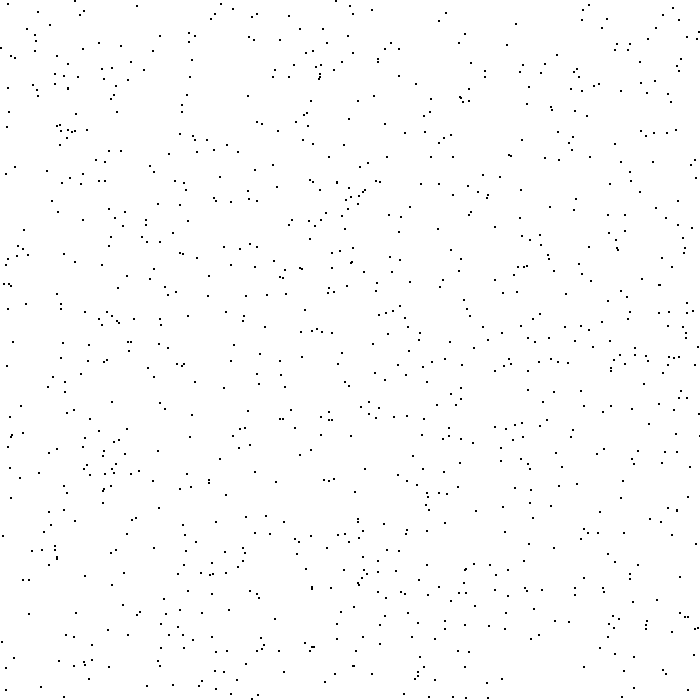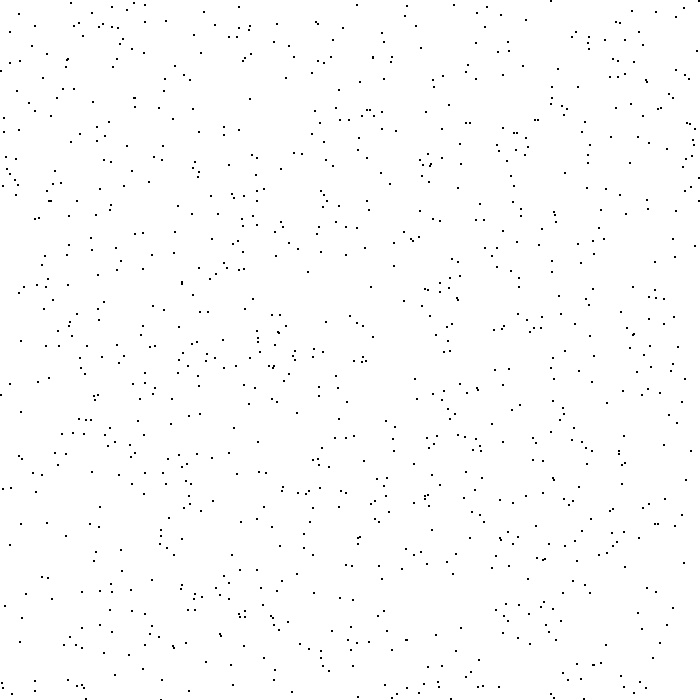So far, they look pretty similar in terms of distribution. Let's try 10,000 hashes: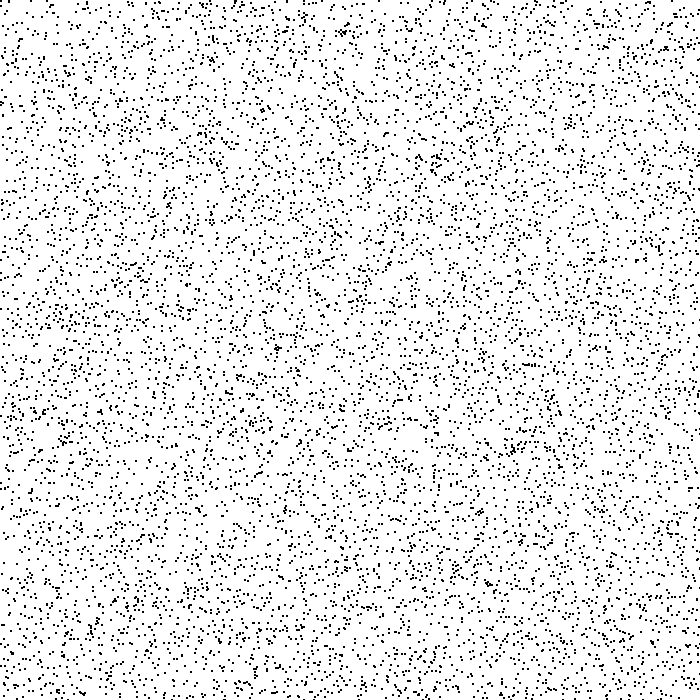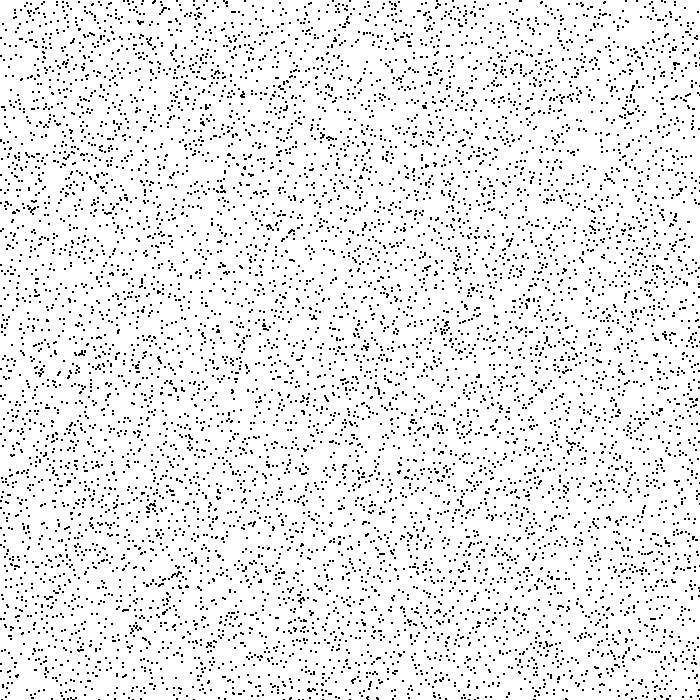Still looking pretty similar in terms of distribution. Let's try 100,000 hashes: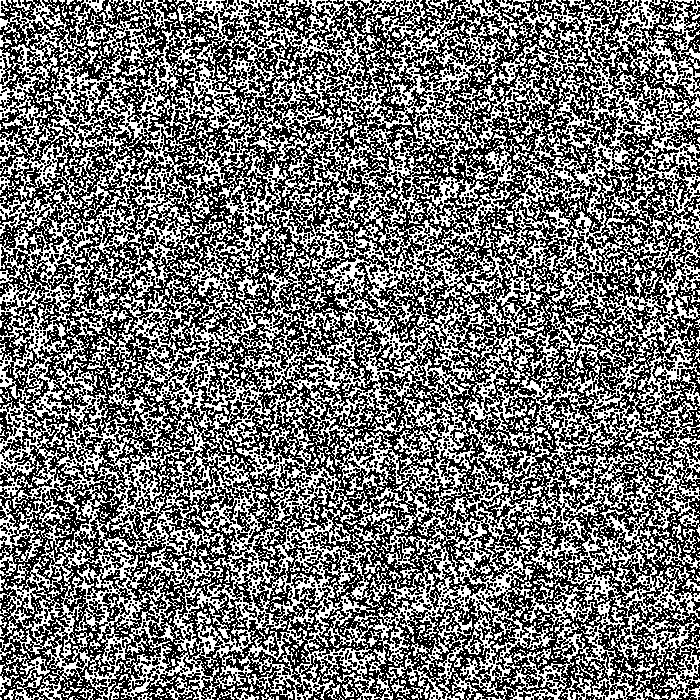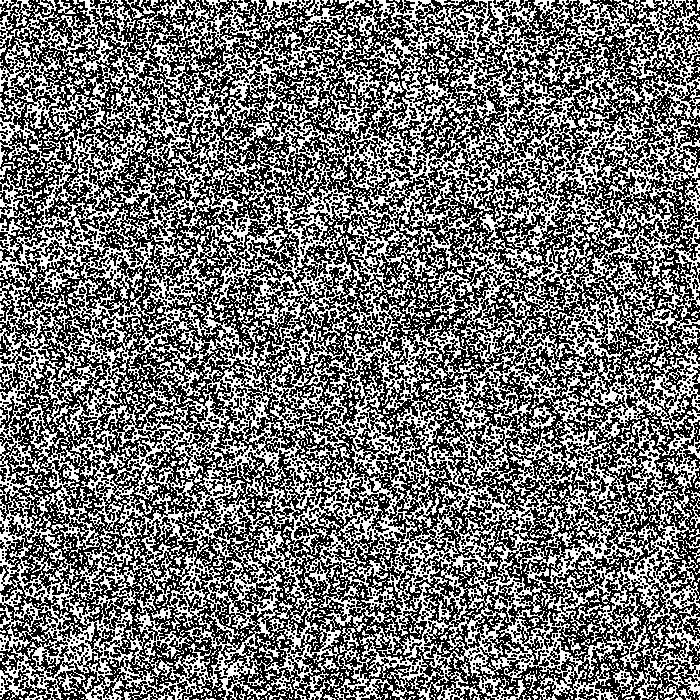And, finally, let's try with 1,000,000 hashes:At this point, our 700 x 700 canvas is essentially covered. Each hash generation appears to have a good distribution across the full space (or, rather, across the modulus space scaled down for our canvas size).

Again, I can's speak to any of this from a mathematical or scientific standpoint; but, to my silly human eyes, the order of the hash inputs does not appear to have a noticeable impact on the distribution and uniqueness of the hash outputs. If there were to be an impact, I believe we would expect to see the two different canvases start to diverge in terms of coverage.

For completeness, here's my full ColdFusion (Lucee CFML) script:

``````<cfscript>

setting
requestTimeout = 300
;

// Every hash will include an incremented counter value.
counter = randRange( 0, 2057, "sha1prng" );
// Every hash will include a hash of the SERVER scope, which includes product and
// machine information such as the Host Name and the MAC address.
fingerprint = hash( serializeJson( server ), "sha-256" );
// Every hash will include a random selection of bytes, generated per hash.
secureRandom = createObject( "java", "java.security.SecureRandom" )
.init()
;

// Cache the Java class instances for use in the hashing functions.
MessageDigestClass = createObject( "java", "java.security.MessageDigest" );
BigIntegerClass = createObject( "java", "java.math.BigInteger" );

// ------------------------------------------------------------------------------- //
// ------------------------------------------------------------------------------- //

// We're going to draw dots (1x1 rectangles) to two different canvas. Each canvas will
// represent the results of hashing the SAME INPUTS in a DIFFERENT ORDER.
canvasSize = 700;
canvasOne = createCanvas( canvasSize )
canvasTwo = createCanvas( canvasSize );

loop times = 1000000 {

// Each call for rand-ints will return a 2-tuple in which each value is the result
// of hashing the SAME INPUTS in a DIFFERNT ORDER. While the order of hash inputs
// will clearly affect the absolute value, the goal here is to see if the input
// order affects the CHARACTERISTICS of the value (ie, its randomness and general
// distribution in a given space).
xCoords = randInts();
yCoords = randInts();

canvasOne.drawRect( xCoords[ 1 ], yCoords[ 1 ], 1, 1, true );
canvasTwo.drawRect( xCoords[ 2 ], yCoords[ 2 ], 1, 1, true );

}

canvasOne.writeToBrowser();
canvasTwo.writeToBrowser();

// ------------------------------------------------------------------------------- //
// ------------------------------------------------------------------------------- //

/**
* I return a 2-tuple of the givens sources of entropy hashed together in two different
* orders.
*
* NOTE: Each hash is converted into a number and fit into the CANVAS SIZE range so
* that it can be used a X/Y coordinate.
*/
public array function randInts() {

var timeBytes = charsetDecode( getTickCount( "nano" ), "utf-8" );
var counterBytes = charsetDecode( counter++, "utf-8" );
var fingerprintBytes = charsetDecode( fingerprint, "utf-8" );
var randomBytes = nextBytes( 32 );

// Return a 2-tuple with the same inputs hashed in a different order.
return([
hashInputs([ timeBytes, counterBytes, fingerprintBytes, randomBytes ]),
hashInputs([ randomBytes, fingerprintBytes, counterBytes, timeBytes ]),
]);

}

/**
* I hash the given inputs, convert them to a number, and then modulus them into the
* canvas size.
*/
public numeric function hashInputs( required array inputs ) {

var digest = MessageDigestClass.getInstance( "sha-256" );

for ( var value in inputs ) {

digest.update( value );

}

var remainder = BigIntegerClass
.init( digest.digest() )
.remainder( BigIntegerClass.valueOf( canvasSize ) )
;

return( abs( remainder ) );

}

/**
* I return an array of random bytes using the Secure Random class.
*/
public array function nextBytes( required numeric length ) {

// Create a Java byte array of the desired length.
var bytes = [];
arrayResize( bytes, length );
arraySet( bytes, 1, length, 0 );

// Write the random bytes into the byte array.
var javaBytes = javaCast( "byte[]", bytes );
secureRandom.nextBytes( javaBytes );

return( javaBytes );

}

/**
* I create and configure a ColdFusion image of the given size.
*/
public any function createCanvas( required numeric size ) {

var canvas = imageNew( "", size, size, "rgb", "ffffff" );
canvas.setDrawingColor( "000000" );
canvas.setAntialiasing( "off" );

return( canvas );

}

</cfscript>
``````

This should allow me to simplify parts of my CUID2 for ColdFusion algorithm. And, perhaps make it faster.

Want to use code from this post? Check out the license.